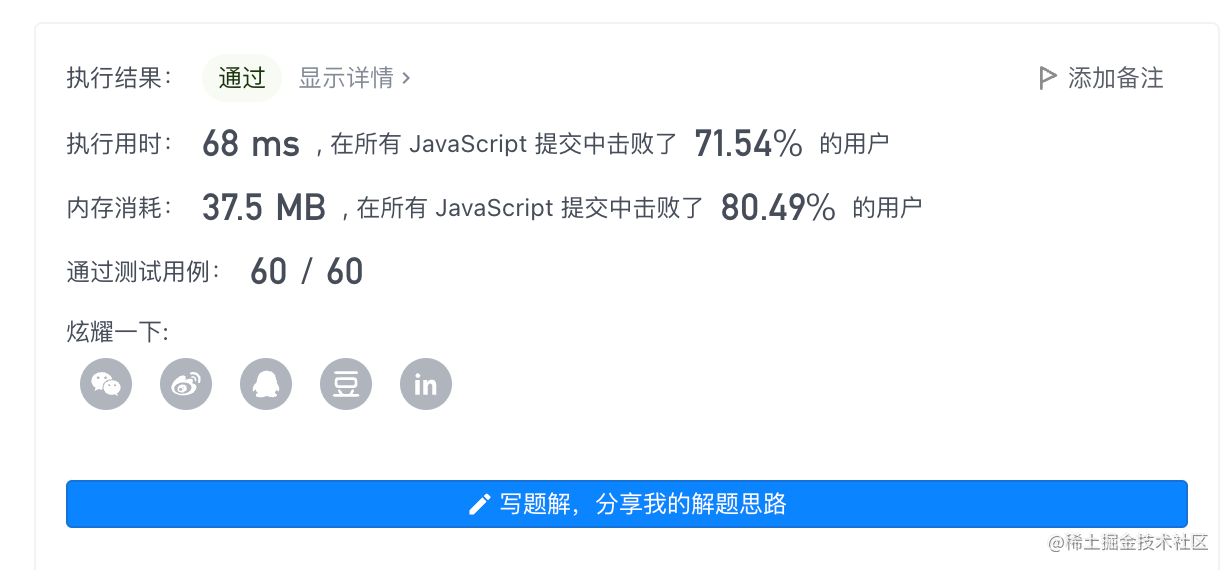2022-11-1700

1. 题目

# 1. 题目

``````输入： solution="RGBY",guess="GGRR"

``````

``````len(solution) = len(guess) = 4
solution和guess仅包含"R","G","B","Y"这4种字符
``````

# 解：哈希法

1. 遍历一遍solution 先求“猜中”了几次
2. 再求“伪猜中”了几次，用一个哈希数组存储伪猜中的字母对应的次数，与solution这个字母出现的次数相比，如果小了就+1
3. 因为题目要求伪猜中不包含猜中，所以减一下
```javascript```var masterMind = function(solution, guess) {
const hash = {
Y: 0,
B: 0,
G: 0,
R: 0
}
for(let i = 0; i < 4; i++) {
const num = solution.split(guess[i]).length - 1

if(solution[i] === guess[i]) {
}
if(num && hash[guess[i]] < num) {
hash[guess[i]]++
}
}
};
``````复杂度分析：

• 时间复杂度：`O(n)`
• 空间复杂度：`O(n)`